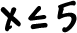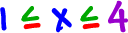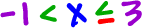TRY IT:

Graphon a number line.

Graphon a number line.This means that x can be 1... or x can be 4 ... or x can be any number between 1 and 4 ... Like 2 or 3.01459.

One way to write this interval is(read as "x is greater than or equal to 1 and less than or equal to 4")

So, how would we graph this on a number line?